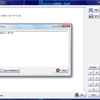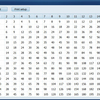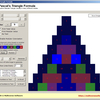Mathematics

Mathematics softwareFalco Calculator

Falco Calculator is a useful program for education. It allows to calculate entered meanings of variables which are introduced as a formula.Magic Box

Magic Box is a collection of applications. In it you will find some popular jokes and puzzles such as magic square, magic eye, latent image fading dollar and math transformation. W ...Times Table

Create and print custom time tables of different values and sizes.Pascal's Triangle Formula

Explore Pascal's Triangle visually. Pascal's Triangle Formula lets you zoom in and modify many properties of the triangle in a visual way. Pascal's Triangle is a famous and simple ...